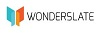# MATHEMATICS AND STATISTICS – PART 1 For SCIENCE & ARTS – PRAGATI EASY GUIDES – As per 2020 Syllabus for Class 12th – Junior College

230.00

 Authors Name Maruti D. Bhagat, Anagha R. Medhekar Edition First Publishing Year June 2020 Pages 230 ISBN 9789389944778 Language English Buy E-Book  (PDF Version)[For IOS , Android & Desktop - MCQ Generator Included]

SKU: P0047

For E-Books purchased from Kopykitab.com

For E-Books purchased from Amazon

##### Description

1. Mathematical Logic

• Identify statement in logic and truth value of it. | • Combine two or more statements. | • Construct the truth table and examine logical equivalence of statement patterns. | • Find dual and negation of statement pattern. | • Study the applications of logic to switching circuits.

2. Matrices

• Identify orders and types of matrices. | • Perform basic algebraic operations on matrices. | • Find the inverse of a matrix using elementary transformation and adjoint method. | • Solve the system of linear equations using matrices.

3. Trigonometric Functions

• Understand and write trigonometric equation. | • Find the principal and general solution of a trigonometric equation. | • Solve triangle by using sine rule, cosine rule and projection rule and find area of a triangle. | • Understand inverse trigonometric functions with domain and range.

4. Pair of Straight Lines

• Write and interpret the combined equation of two straight lines in plane. | • Find the plane of intersection of two lines and calculate the acute angle between them. | • Study the general second degree equation in x and y with reference to homogeneous part of it.

5. Vectors

• Understand scalars and vectors and algebra of vectors. | • Write vectors of 2 or 3 dimensions, understand the scalar and vector products. | • Study applications of vectors to area of triangle, work done by a force, moment of a force. | • Interpret scalar triple product and its applications.

6. Line and Plane

• Find different forms of equation of line. | • Find angle between two intersecting planes. | • Find the angle between a line and a plane. | • Find condition for perpendicularity and parallelness of planes. | • Calculate distance of a point from a plane. | • Find equation of a plane in different forms. | • Find angle between two intersecting planes. | • Find the angle between a line and a plane.

7. Linear Programming

• Understand linear equations in one and two variables. | • Find graphical solution of linear inequation. | • Understand meaning and formulation of L.P.P. | • Find solution of L.P.P. by graphical methods.

Features of the Book :

(1) We have tried our best to give detailed solutions to almost all the problems. Elaborate explanation is provided wherever it is necessary.

(2) We have also given parallel MCQ’s, problems and examples at the end of each chapter for practice and improvement of the students.
Before solving the problems, students are advised to memorise various formulae that are required.